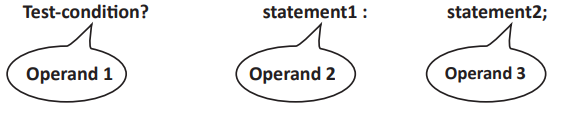##### CCC Online Test

Qus. What are Arithmetic operators? What are the different forms of arithmetic operators?

Ans. Arithmetic operators are used to evaluate mathematical expressions. Arithmetic operators are a form of operator that may either by a Unary Operator or a Binary Operator.

Qus. State the different types of binary arithmetic operators. Also state their hierarchy of operations.

Ans These are operators that work on two operands. The operator is placed between the operands. The symbols that are used as binary arithmetic operators are:

- For performing subtraction.

* For performing multiplication.

/ For performing division.

% For getting the remainder when divided.

Hierarchy of Arithmetic Operators

1. First Brackets/Parenthesis: In Java the expression in bracket is done first. However brackets in an expression should only be first bracket ( ). Nested brackets can also be used, but in that case the expressions in the innermost bracket are done first and then the subsequent outerlevel brackets. Eg. (5+6*(7-3)) will give 29. Notice that only first-bracket is used.

2. Then /, *, or % whichever comes first while evaluating from left to right will get evaluated first.

3. Then + or – whichever comes first while evaluating from left to right will get evaluated first..

Qus. What is increment/decrement operator used for? Explain with the help of an example.

Ans. The increment/decrement operator is used to increase/decrease the value of a variable by 1. For example a++; increases the value of the variable a by 1, similarly a--; decreases the value of the variable a by 1.

Qus. What are short hand operators? Explain with an example.

Ans. Java provides special operators that can be used to combine an arithmetic operation with an assignment. For example, the expression,

a = a+4;

can be written as,

a += 4;

As this operator is a shorter way of expressing an expression it is also sometimes called Short hand operators.

Qus. Name the logical operators used in Java.

 Logical Operator Name Behaviour && AND operator Checks whether the conditions preceding and succeeding it is true or not. || OR operator Checks whether either of the conditions preceding and succeeding it is true or not. ! NOT operator Just negates the logic of the condition succeeding it to check for its validity.

Qus. Name the relational operators along with their usage in Java.

Ans.

 Relational operator Usage > Greater than < Less than >= Greater than or equal to <= Less than or equal to = = Equal to != Not equal to

Qus. What is Operator Associativity? What is the operator associativity of prefix/postfix increment/ decrement operators?

Ans. Operator Associativity is a property that determines how operators of the same precedence are grouped in the absence of parentheses; i.e., in what order each operator is evaluated.

 Operator Description Associativity + + Post-Increment left to right − − Post-Increment left to right + + Pre-Increment right to lef − − Pre-Increment right to lef

Qus. Give the general syntax of conditional operator and also label its operands.

Ans. It has the following general syntax:Qus. What is an expression?

Ans. An expression is a construct that is made up of variables, operators and method invocations, which are constructed according to the syntax of the language that evaluates to a single value.